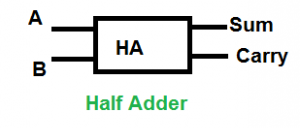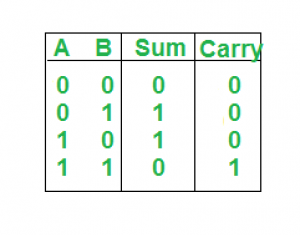Open in App
Not now

# Python program to implement Half Adder

• Last Updated : 21 Aug, 2021

Prerequisite: Half Adder in Digital Logic

Given two inputs of Half Adder A, B. The task is to implement the Half Adder circuit and Print output i.e sum and carry of two inputs.

Half Adder: A half adder is a type of adder, an electronic circuit that performs the addition of numbers. The half adder is able to add two single binary digits and provide the output plus a carrying value. It has two inputs, called A and B, and two outputs S (sum) and C (carry).Logical Expression:

```Sum = A XOR B
Carry = A AND B ```

Truth Table:Examples:

Input : 0 1

Output: Sum=1, Carry=0

Explanation: According to logical expression Sum=A XOR B  i.e 0 XOR 1 =1 , Carry=A AND B  i.e 0 AND 1 =0

Input : 1 1

Output: Sum=0, Carry=1

Explanation: According to logical expression Sum=A XOR B  i.e 1 XOR 1 =0 , Carry=A AND B  i.e 1 AND 1 =1

Approach:

• We take two inputs A and B.
• XOR operation on A and B gives the value of the sum.
• AND operation on A and B gives the value of Carry.

Below is the implementation.

## Python3

 `# Function to print sum and carry``def` `getResult(A, B):``  ` `    ``# Calculating value of sum``    ``Sum` `=` `A ^ B``    ` `    ``# Calculating value of Carry``    ``Carry ``=` `A & B``    ` `    ``# printing the values``    ``print``(``"Sum "``, ``Sum``)``    ``print``(``"Carry"``, Carry)`  `# Driver code``A ``=` `0``B ``=` `1` `# passing two inputs of halfadder as arguments to get result function``getResult(A, B)`

Output:

```Sum  1
Carry 0```
My Personal Notes arrow_drop_up